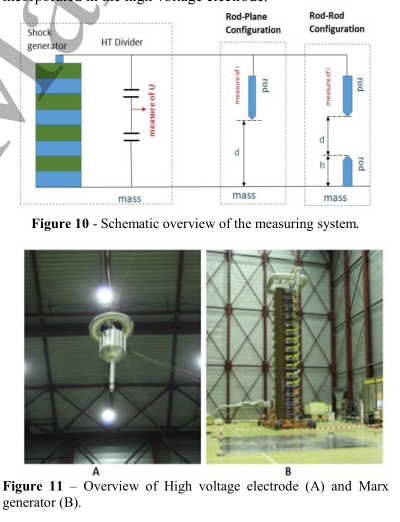# 2. 正极性棒棒间隙放电发展步骤

(1) HV 电极上第一条电晕流注的开始。 (2)根据高压电极的特性和电压波形，存在一段以电极尖端不发光为特征的持续时间不定的时期，即暗期。 (3) 随着电压的增加，电离恢复，放电通道，领导者，向相反的电极发展。 领导者的头部由一个类似于在电极（电晕领导者）上观察到的冠冕组成。 放电的亮度主要是由于领导者的头部，领导者的通道是微弱的。 领导者所走的路径非常曲折，与初始静电场的力线不符。 引导器的引导速度约为 2 cm/μs，其直径约为几毫米。 根据所施加电压的值和形状以及间隙的长度，先导可以停止或到达对面的电极。 （4） 在构成正极引线电晕的拖缆到达负极后，可以注意到从该电极开始的第二个引线的形成以几乎呈指数增长的速度在间隙中向正极前进，并连接正极引线。这种机制称为最终跳跃，如图1所示，其中L+和L-分别是负引线和正引线的轴向长度。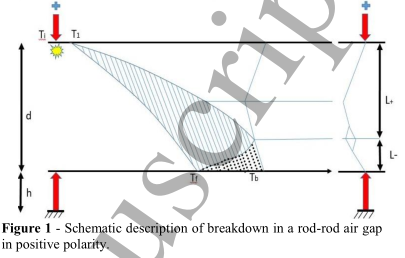# 3. 放电的棒-棒间隙模型

## 3.1 总述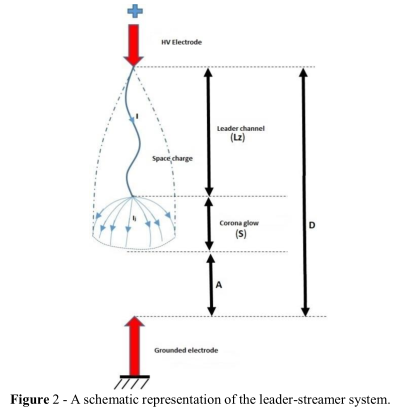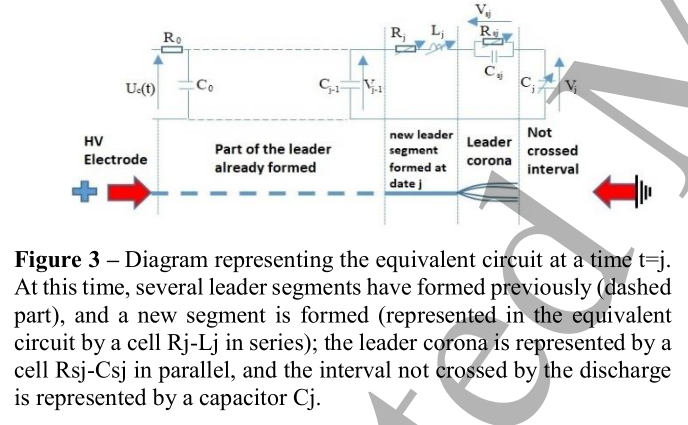## 3.2 计算等效电极对导线和电场的影响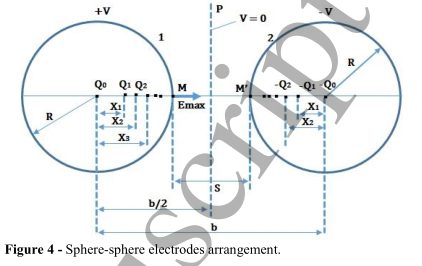$E _ { P } = 1.8 \frac { U _ { j } } { ( D - L _ { z } ) } \frac { R _ { e } + 0.5 ( D - L _ { z } ) } { R _ { e } } \tag{1}$

$R _ { e } = \frac { U _ { j } * 0.25 } { U _ { j 0 } ( 1 + \frac { 3.5 } { L } ) } + R _ { e } e ^ { - ( \frac { L _ { z } } { 13.6 R _ { i } } ) } \tag{2}$

𝐸𝑐取决于电极的形状。对于球形电极，它是如:

$E _ { c } = [ 1 + \frac { 0.0436 } { \sqrt { R _ { e } \delta } } ] \tag{3}$

## 2.2 通过等效电路建模先导放电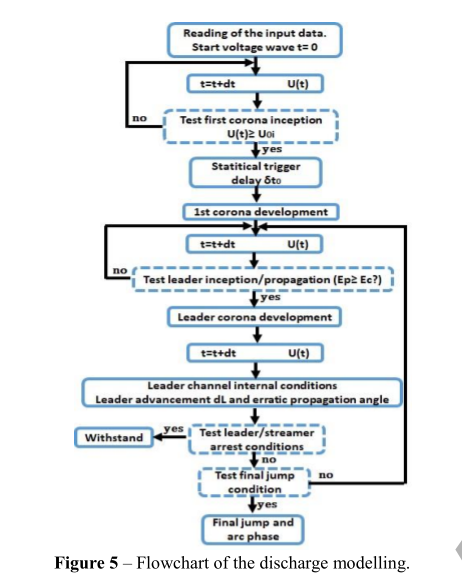$P ( \varphi ) = \frac { | \operatorname { sin } ( \varphi ) | } { 2 a [ 1 - \operatorname { exp } ( \frac { 2 } { a } ) ] } e ^ { [ \frac { \operatorname { cos } ( \varphi ) - 1 } { a } ] } \tag{4}$

## 3.4 仿真

$U _ { 50 } = k 1080 \operatorname { ln } ( 0.46 D + 1 ) \tag{5}$

$| \frac { U 50 _ { \text { comp } } - U 50 _ { \text { criepi } } } { U 50 _ { \text { comp } } } | = 0.039 \tag{6}$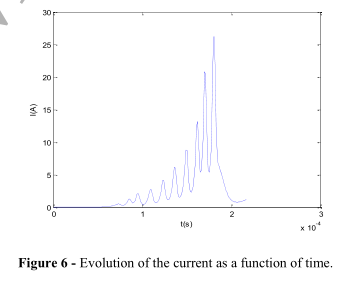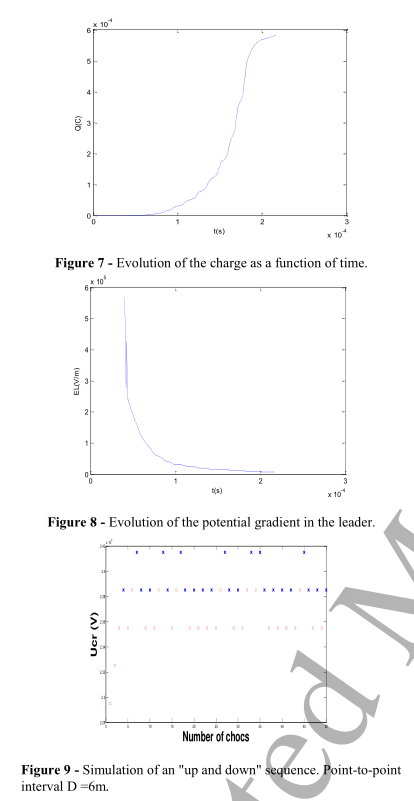# 4. 试验

## 4.1 简介

2015 年 10 月至 2016 年 6 月期间，我们在 Renardiéres 高压实验室 (EDF) 进行了两次介电测试活动； 主要目的是测量放电期间引线内电流的演变，以及确定 U50 电压和棒平面和棒棒间隔的 k 因子。 考虑的电极间隙 d 在 2 到 5 米之间。 针对雷电脉冲研究每个间隙的行为，并针对批判性冲动。 在实验测量过程中，我们遇到了一些困难，主要困难是：

## 4.2 试验装置介绍